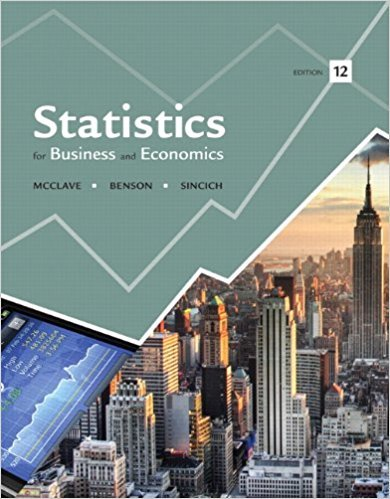×
Log in to StudySoup
Get Full Access to Statistics For Business And Economics - 12 Edition - Chapter 8 - Problem 3e
Join StudySoup for FREE
Get Full Access to Statistics For Business And Economics - 12 Edition - Chapter 8 - Problem 3e

Already have an account? Login here
×
Reset your password

# In order to compare the means of two populations,ISBN: 9780321826237 51

## Solution for problem 3E Chapter 8

Statistics for Business and Economics | 12th Edition

• Textbook Solutions
• 2901 Step-by-step solutions solved by professors and subject experts
• Get 24/7 help from StudySoup virtual teaching assistantsStatistics for Business and Economics | 12th Edition

4 5 1 432 Reviews
16
4
Problem 3E

Problem 3E

In order to compare the means of two populations, independent random samples of 400 observations are selected from each population, with the following results:

 Sample 1 Sample 2= 5,275= 5,240 s1 = 150 s2 = 200

a. Use a 95% confidence interval to estimate the difference between the population means (µ1-µ2) Interpret the confidence interval.

b. Test the null hypothesis H0: (µ1-µ2) = 0 versus the alternative hypothesis Ha: Ha: (µ1-µ2) ≠ 0 Give the p-value of the test, and interpret the result. c. Suppose the test in part b were conducted with the alternative hypothesis Ha: Ha: (µ1-µ2) > 0 How would your answer to part b change?

d. Test the null hypothesis H0: (µ1-µ2) = 25versus the alternative Ha: Ha: (µ1-µ2) ≠ 25.Give the p-value, and interpret the result. Compare your answer with that obtained from the test conducted in part b.

e. What assumptions are necessary to ensure the validity of the inferential procedures applied in parts a–d?

Step-by-Step Solution:

Solution:

Step 1 of 6:

It is given that two samples of size 400 each are drawn from two independent populations to compare their means.

The mean and the standard deviations of the two samples are given.

 Sample 1 Sample 2=5,275=5,240=150=200

Using this data we need to find the required values.

Step 2 of 6

Step 3 of 6

##### ISBN: 9780321826237

Statistics for Business and Economics was written by and is associated to the ISBN: 9780321826237. This textbook survival guide was created for the textbook: Statistics for Business and Economics , edition: 12. This full solution covers the following key subjects: test, hypothesis, alternative, interpret, part. This expansive textbook survival guide covers 15 chapters, and 1631 solutions. The full step-by-step solution to problem: 3E from chapter: 8 was answered by , our top Business solution expert on 07/21/17, 05:42AM. The answer to “In order to compare the means of two populations, independent random samples of 400 observations are selected from each population, with the following results:Sample 1Sample 2 = 5,275 = 5,240 s1 = 150s2 = 200a. Use a 95% confidence interval to estimate the difference between the population means (µ1-µ2) Interpret the confidence interval.________________b. Test the null hypothesis H0: (µ1-µ2) = 0 versus the alternative hypothesis Ha: Ha: (µ1-µ2) ? 0 Give the p-value of the test, and interpret the result. c. Suppose the test in part b were conducted with the alternative hypothesis Ha: Ha: (µ1-µ2) > 0 How would your answer to part b change?________________d. Test the null hypothesis H0: (µ1-µ2) = 25versus the alternative Ha: Ha: (µ1-µ2) ? 25.Give the p-value, and interpret the result. Compare your answer with that obtained from the test conducted in part b.________________e. What assumptions are necessary to ensure the validity of the inferential procedures applied in parts a–d?” is broken down into a number of easy to follow steps, and 156 words. Since the solution to 3E from 8 chapter was answered, more than 314 students have viewed the full step-by-step answer.

Unlock Textbook Solution

Enter your email below to unlock your verified solution to:

In order to compare the means of two populations,